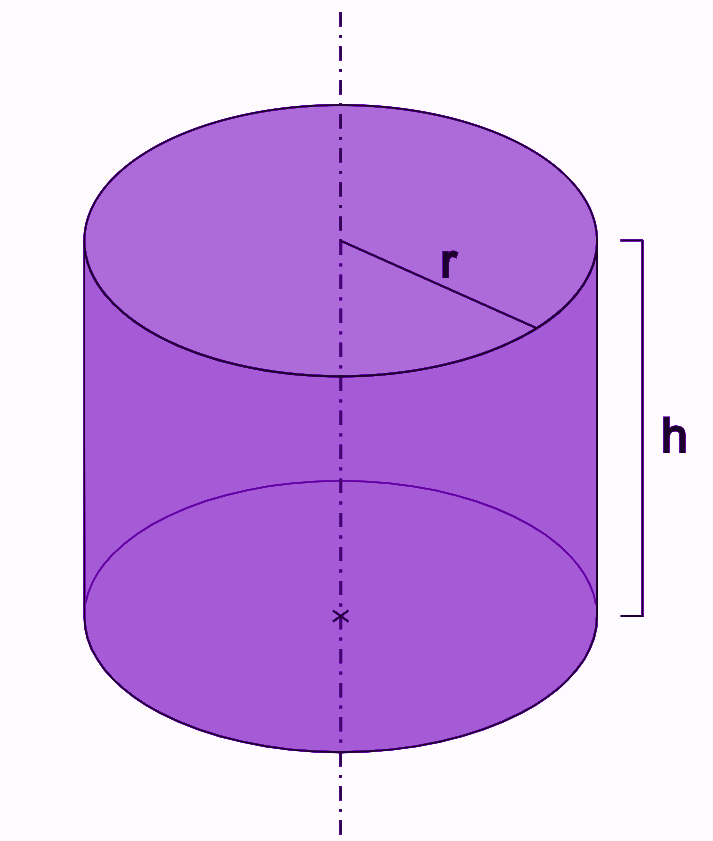# Right Circular Cylinder

A right circular cylinder is a cylinder that has a closed circular surface having two parallel bases on both the ends and whose elements are perpendicular to its base. It is also called a right cylinder. All the points lying on the closed circular surface is at a fixed distance from a straight line known as the axis of the cylinder. The two circular bases of the right cylinder have the same radius and are parallel to each other. It is one such geometric shape which is used frequently in real life. Basically, to derive the formulas for area and volume of the cylinder, the right cylinder is considered. There is also one more type of cylinder known as an oblique cylinder in 3D geometry, which is a special case.

## Right Circular Cylinder Definition

A cylinder whose bases are circular in shape and parallel to each other is called the right circular cylinder. It is a three-dimensional shape. The axis of the cylinder joins the centre of the two bases of the cylinder. This is the most common type of cylinder used in day to day life. Whereas the oblique cylinder is another type of cylinder, which does not have parallel bases and resembles a tilted structure.In the above figure, r is the radius of the circular bases and h is the height of the right cylinder.

### Properties of Right Circular Cylinder

You must have learned about the properties of the cylinder before. Here, let us discuss the right circular cylinder properties.

• The line joining the centres of the circle is called axis.
• When we revolve a rectangle about one side as the axis of revolution, a right cylinder is formed.
• The section obtained on cutting a right circular cylinder by a plane, which contains two elements and parallels to the axis of the cylinder is the rectangle.
• If a plane cuts the right cylinder horizontally parallel to the bases, then its a circle.

## Right Circular Cylinder Formula

A surface, which is generated by a line which intersects a fixed circle and is perpendicular to the plane of the circle is said to be a right circular cylinder. A right circular cylinder has two circular bases which are of the same radius and are parallel to each other. The formulas for surface area, curved or lateral surface area and volume of the right cylinder are discussed here.

### Curved Surface Area

The surface area of a closed right circular cylinder is the sum of the area of the curved surface and the area of the two bases. The curved surface that joins the two circular bases is said to be the lateral surface of the right circular cylinder.

 Lateral or Curved Area = 2 π r h square units

### Total Surface Area

The sum of the lateral surface area and the base area of both the circles will give the total surface area of the right circular cylinder.

 TSA = 2 π r (h + r) square units

### Volume

The volume of a right cylinder is given by the product of the area of the top or bottom circle and the height of the cylinder. The volume of a right cylinder is measured in terms of cubic units.

Volume = Area of the circular bases x Height of the Right Cylinder

 Volume = πr2 h

### Solved Examples

Let us solve some problems based on the formulas of the right circular cylinder.

Q.1: Find the volume of a right cylinder, if the radius and height of the cylinder are 20 cm and 30 cm respectively.

Solution: We know,

Volume of a right cylinder = πr2 h cubic units

Given, r = 20 cm h = 30 cm

Therefore, using the formula, we get;

Volume = 3.14 × 202 × 30

= 3.14 × 20 × 20 × 30

= 37680

Hence, the volume of the given right cylinder is 37680 cm3.

Q.2: The radius and height of a right cylinder are given as 5 m and 6.5 m respectively. Find the volume and total surface area of the right cylinder.

Solution: Given that, r = 5 m h = 6.5 m

We know, by the formula,

Volume of a right cylinder = πr2 h cubic units

Therefore,

Volume = 3.14 × 52 × 6.5

= 3.14 × 25 × 6.5

= 510.25

Hence, the volume of the given right cylinder is 510.25 cubic m.

Now we know again, the total surface area of the right cylinder is given by;

TSA = Area of circular base + Curved Surface Area

TSA = 2 π r (h + r) square units

By putting the values of radius and height, we get;

TSA = 2 x π x 5 (6.5 + 5)

TSA = 2 x 3.14 x 5 x 11.5

TSA = 361.1 sq.m

Hence, the total surface area of the given right cylinder is 361.1 m2.

Test your knowledge on Right Circular Cylinder